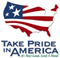# Evaluation of Pier-Scour Equations for Coarse-Bed Streams

### By Katherine J. Chase and Stephen R. Holnbeck U.S. Geological Survey Scientific Investigations Report 2004-5111

In cooperation with the
Montana Department of Transportation

### Abstract

Streambed scour at bridge piers is among the leading causes of bridge failure in the United States. Several pier-scour equations have been developed to calculate potential scour depths at existing and proposed bridges. Because many pier-scour equations are based on data from laboratory flumes and from cohesionless silt- and sand-bottomed streams, they tend to overestimate scour for piers in coarse-bed materials. Several equations have been developed to incorporate the mitigating effects of large particle sizes on pier scour, but further investigations are needed to evaluate how accurately pier-scour depths calculated by these equations match measured field data.

This report, prepared in cooperation with the Montana Department of Transportation, describes the evaluation of five pier-scour equations for coarse-bed streams. Pier-scour and associated bridge-geometry, bed-material, and streamflow-measurement data at bridges over coarse-bed streams in Montana, Alaska, Maryland, Ohio, and Virginia were selected from the Bridge Scour Data Management System. Pier scour calculated using the Simplified Chinese equation, the Froehlich equation, the Froehlich design equation, the HEC-18/Jones equation and the HEC-18/Mueller equation for flood events with approximate recurrence intervals of less than 2 to 100 years were compared to 42 pier-scour measurements. Comparison of results showed that pier-scour depths calculated with the HEC-18/Mueller equation were seldom smaller than measured pier-scour depths. In addition, pier-scour depths calculated using the HEC-18/Mueller equation were closer to measured scour than for the other equations that did not underestimate pier scour. However, more data are needed from coarse-bed streams and from less frequent flood events to further evaluate pier-scour equations.

### Contents

Abstract
Introduction
Purpose and scope
Description of pier-scour data used to evaluate equations
Methods of data collection
Comparison of data sets used to develop and evaluate equations
Description of pier-scour equations for coarse-bed streams
Simplified Chinese equation
Froehlich equation
Froehlich design equation
HEC-18/Jones equation
HEC-18/Mueller equation
Evaluation of pier-scour equations for coarse-bed streams
Simplified Chinese equation
Froehlich equation
Froehlich design equation
HEC-18/Jones equation
HEC-18/Mueller equation
Summary statistics for calculated and measured pier scour
Summary and conclusions
References cited

### ILLUSTRATIONS

1. Map showing States from which pier-scour data were compiled
2. Graphs showing comparison of calculated to measured pier scour for five equations
3. Graph showing distribution of residual depth of pier scour

### TABLES

1. Hydrologic and hydraulic data for selected pier-scour sites
2. Summary of pier-scour measurements
3. K1, K2, and K3 correction factors for the HEC-18/Jones equation
4. Summary of scour measurement data used to develop the HEC-18/Mueller equation
5. Comparison of calculated pier scour to measured pier scour from five equations
6. Summary statistics for calculated pier scour for five pier-scour equations and measured pier scour

 AccessibilityFOIAPrivacyPolicies and Notices U.S. Department of the Interior, U.S. Geological Survey Persistent URL: Page Contact Information: Contact USGS Last modified: Thursday, January 10 2013, 06:41:16 PM